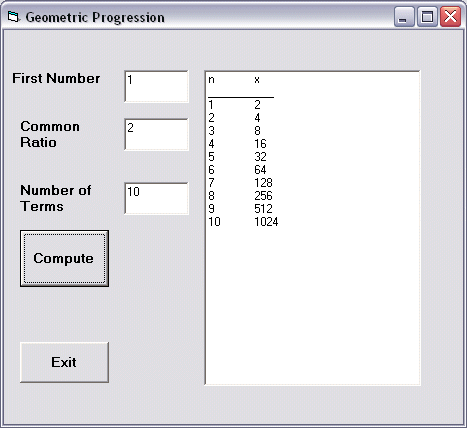# Geometric Progression Generator

This is a Visual Basic program that generates a geometric progression and displays the results in a list box. Geometric progression is a sequence  of numbers where each subsequent number is found by multiplying the previous number by a fixed number. The fixed number is called the common ratio. The common ratio can be negative, an integer, a fraction and any number but it must not be a zero.

The formula to find the nth term of the geometric progression is   arn-1 , where a is the first number and r is the common ratio.

In visual basic, we employs the Do.... Loop Until statement to generate the numbers in a geometric progression.In this program, we need to insert three text boxes for the user to enter the first number, the common ratio and the number of terms. We also need to insert a list box to list the numbers. Besides that, a command button is need for the user to generate the numbers in the geometric progression.

To add the numbers to the list box, we use the AddItem method. The syntax is List1.AddItem n, where n can be any variable. The runtime interface is shown in the figure below.

## The Runtime Interface### The Code

Private Sub cmd_compute_Click()
Dim x, n, num As Integer
Dim r As Single
x = Txt_FirstNum.Text
r = Txt_CR
num = Txt_Terms.Text
List1.AddItem "n" & vbTab & "x"

n = 1
Do
x = x * r
List1.AddItem n & vbTab & x
n = n + 1
Loop Until n = num + 1
End Sub